# Active Low Pass Filter: Design and Applications

Contents

## What is an Active Filter?

An active filter is a type of filter that includes one or more active circuit components such as a transistor or an operational amplifier (Op-Amp). They derive their energy from an external source of energy and use it to increase or amplify the signal output.

Operational amplifiers can also be used to form or change the circuit frequency response by making the filter’s output bandwidth narrower or even wider by generating a more selective output reaction.

An Op-Amp has a high input impedance, a low output impedance and a voltage gain within its feedback loop arising from the mixture of the resistor. Active filters, when used with careful circuit design, generate excellent performance features, very good precision with a steep roll-off and low noise.

## What is an Active Low Pass Filter?

If an active filter permits only low-frequency components and denies all other high-frequency components, then it is termed as an Active Low Pass Filter. Active low pass filters are made up of Op-Amp. The input to the Op-Amp is high impedance signals, which produces a low impedance signal as output.

The performance of the amplifier plays a very important factor when designing an active low pass filter. There are two primary kinds of active low-pass filters namely switched capacitor type and continuous capacitor type. The filters are available from first-order until the eighth order of design.

The circuit’s frequency response will be the same as that of the passive RC filter, except that the passband voltage gain increases the amplitude of the output signal.

The passband begins from 0Hz or DC for a low pass filter and continues at -3dB to the designated cut-off point. Signals are attenuated beyond the cut off frequency. Active low pass filters are grouped according to the order of the filter. We will discuss 1st & 2nd order active low pass filters.

The inverse of a low pass filter is a high pass filter, that permits signals with frequencies higher than the cut-off frequency and blocks all frequencies below this cut-off frequency. There are also bandpass filters, which combine the functionality of low pass filters and high pass filters to only allow frequencies within a specific frequency range.

## First Order Active Low Pass Filter

A first-order active low pass filter is a simplistic filter that is composed of only one reactive component Capacitor accompanying with an active component Op-Amp. A resistor is utilized with the capacitor or inductor to form RC or RL low pass filter respectively. In a passive circuit, the output signal amplitude is smaller than the input signal amplitude.

To surmount this problem, active circuit designs were introduced. When a passive low pass filter is connected to an Op-Amp either in inverting or non-inverting condition, it gives an active low pass filter design. The connection of a simple RC circuit with a single Op-Amp is shown in the image below.

This RC circuit assists in providing a low-frequency signal to the input of the amplifier. The amplifier operates as a unity gain output buffer circuit. This circuit has added input impedance value. The Op-Amp of the circuit has a very low output impedance value, which helps in providing high stability to the filter.

When compared with passive filter, an active filter has an arrangement in which the amplifier is designed as a voltage-follower (Buffer) which gives a DC gain of one. This configuration provides excellent stability to the filter and has a high power gain. The main drawback of this filter is that it has no voltage gain above one, which can be rectified with extra circuit design.

### First Order Active Low Pass Filter with Amplification

As discussed, the above filter design does not provide voltage gain above one. Hence we need to modify the circuit as shown below.

At lower frequencies, the input signals flow directly through the amplifying circuit. When the frequency of the input frequency increases, it is bypassed and made to pass through the capacitor C. This increases the amplitude of the output signal by passband gain.

In a non-inverting amplifier circuit configuration, the measurement of the voltage gain for the filter is given as a ratio of the feedback resistor ( R2 ) divided by its corresponding input resistor ( R3 ) value.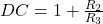### First Order Low Active Pass Filter Inverted Configuration

The inverting low pass filter is designed using IC741, an Op-Amp with 8 pin configuration. In inverting mode, the output of the Op-Amp is 180 degrees out of phase with the input signal. Initially, the Op-Amp has to be supplied with DC power. The amount of DC power will depend on how large the signal will be amplified at the output.

The output is AC signal which has peak-peak value. In this example, we are going to design the circuit for a gain of 10, hence the supply voltage of 12VDC is supplied to V+, pin 7 of the Op-Amp and -12VDC is supplied to V pin 4 of the Op-Amp. This is termed inverted Op-Amp configuration and the output signal will be inverted than the input signal.

### First Order Low Active Pass Filter Non-Inverted Configuration

In this configuration also IC741 Op-Amp is used. But, here the input signal and output signal will be in phase with each other. RC which forms the low pass filter part is the first half of the circuit.

Then the signal is passed through the Op-Amp, where the signal gets amplified by a voltage gain that is proportional to resistors R2 and R1. In this configuration the external impedance makes no effect on the reactance of the capacitor, thus the stability improved.

### First Order Low Pass Filter Voltage Gain

The frequency components are used to obtain the voltage gain of the filter.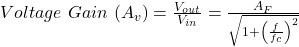where,

Vin is the input voltage
Vout is the output voltage
Af is the passband gain of the filter (1+R2/R1)
f is the frequency of the input signal in Hertz
fc is the cutoff frequency in Hertz

When the frequency is increased, then the gain is decreased by 20 dB. The operation of an active low pass filter can be checked from the above equation of frequency gain. Let f be the operating frequency and fc be the cutoff frequency.

At low frequency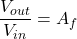When operating frequency is equal to cut off frequency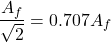And at high frequency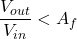From the above equations, it is seen that at low frequencies the gain of the circuit is equal to the maximum value of gain. Whereas at high frequencies condition, the gain of the circuit is very much lesser than the maximum gain Af. When the operating frequency is equal to cut off frequency, the gain is equal to 0.707 Af. In these filter circuits, the quantitative value (magnitude) of the passband gain is expressed in decibels or dB which is a function of the voltage gain.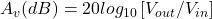### First Order Active Low Pass Filters Transfer Function

The transfer function is also known as systems function or network function of the control system. It is expressed as a mathematical function. When used in frequency domain analysis of a system, it utilizes transform methods like Laplace transform. Filters work on signal frequency.

Thus, analytical and graphical descriptions using the frequency domain are the most potent instruments to describe the conduct of filters. Thus, frequency domain equations and curves of gain vs. frequency and phase vs. frequency are commonly used.

It requires their mathematical description in terms of the system’s transfer function to study the frequency domain of networks. The voltage transfer function is the proportion of the Laplace transforms of the output and input signals for a particular scheme as shown below.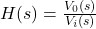Where V0(s) and Vi(s) are the output and input voltages and s is the complex Laplace transform variable.

A single-pole low pass filter is designed for low-frequency applications by connecting a resistor and a capacitor as shown below.

The transfer function of the above circuit can be given as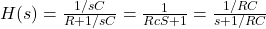Now, in the above equation, the laplace constants are replaced with its equivalent value in frequency domain.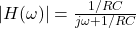The absolute value of transfer function is defined as magnitude or volatge gain and it can be represented as shown below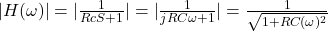The frequency at which the resistance is equal to the impedance of the capacitor, it is said to be at critical frequency which is given as below.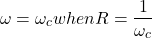Therefore,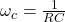After proper substituion into this equation, we get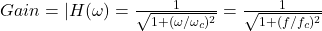The phase shift of the filter is given by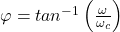The cut off frequency and phase-shift of the filter can be calculated as follows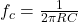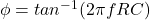Similar filter configuration can be obtained for nth order, when RC stages are cascaded together.

### First Order Active Low Pass Filter Design And Example

Design a non-inverting active low pass filter circuit that has a gain of ten at low frequencies, a high-frequency cut-off or corner frequency of 175Hz and an input impedance of 20KΩ.

The voltage gain of the non-inverting amplifier is given as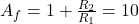Now assume the value of R1 to be 1KΩ and calculate the value R2 from the above equation.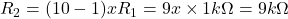Hence for a voltage gain of 10, values of R1 and R2 are 1KΩ and 9KΩ respectively. Gain in dB is given as 20LogA = 20Log10 = 20dB

Now we are given with the cut-off frequency value as 175Hz and input impedance value as 20KΩ. By substituting these values in the equation and value of C can be calculated as follows.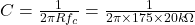= 45.47nF

Thus the final design of filter and its equivalent frequency response curve is shown below.

## Active Low Pass Filter Circuit

A typical circuit for an active low pass filter is given below:

## Active Low Pass Filter Frequency Response Curve

The frequency response curve for an active low pass filter is given below:

## Non-Inverting Amplifier Filter

A simple non-inverting amplifier filter is given below:

## Inverting Amplifier Filter

An equivalent inverting amplifier filter is given below:

## Second Order Active Low Pass Filter

Second-Order Filters are also attributed to as VCVS filters since Op-Amp used here is Voltage Controlled Voltage Source Amplifier. This is another important type of active filter used in applications.

The frequency response of the second-order low pass filter is indistinguishable to that of the first-order type besides that the stopband roll-off will be twice the first-order filters at 40dB/decade. Consequently, the design steps wanted of the second-order active low pass filter are identical. A simple method to get a second-order filter is to cascade two first-order filters.

When filter circuits are cascaded into higher-order filters, the filter’s overall gain is equal to the product of each stage. Active second-order (two-pole) filters are essential because they can be used to design higher-order filters. Filters with an order value can be built by cascading first and second-order filters.

### Second Order Active Low Pass Filter Voltage Gain

The gain of the second-order filter is a product of gain of ‘n’ stages that are cascaded together. For example, if two first-order filters are cascaded, the gain of the filter will be as follows.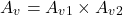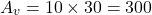If the gain of both stages are given in dB, then the total gain is calculated by adding both gains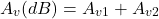### Second Order Active Low Pass Filter Cutoff Frequency

The cut-off frequency equation is given as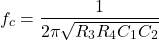When R3 = R4 = R and C1=C2=C then the cut-off frequency will be given asThe gain at the cut-off frequency for the first stage of filter is -3dB. For second order filter, combining the gain of two first order filters, the total gain will be -6dB.

### Second Order Active Low Pass Filter Design And Example

Assume Rs1 = Rs2 = 15KΩ and capacitor C1 = C2 = 100nF. The gain resistors are R1=1KΩ, R2= 9KΩ, R3 = 6KΩ, and R4 =3KΩ. Design a second-order active low pass filter with these specifications.

The cut-off frequency is given as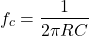(1)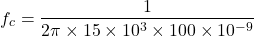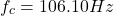The gain of first stage amplifier is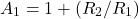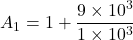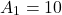The gain of second stage amplifier is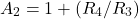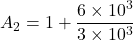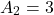Total Gain of the filter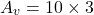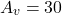The total gain in dB

(2)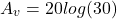(3)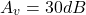The gain at cut-off frequency is

(4)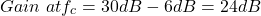## Active Low Pass Filter Applications

These filters are used predominantly in electronics application like in speakers and subwoofers. They act as filter in speakers and as inputs for subwoofers. They play a major role in design of audio amplfiers and equalizers also. When you use analog to digital converters, these filters are used as anti-aliasing filters to control the signals. When it comes to acoustics and sound, the filter is used to stop the high frequency signals from transmitting sound to prevent echoes.

### Active Filtering in Automotive Audio Applications

Operational amplifiers are one of the automotive audio circuits ‘ most popular construction blocks. To boost audio efficiency, many developers choose to integrate Op-Amps into their automotive audio circuits. Active filters eliminate the possibility of undesired interference with the audio signal. Filtering is essential for helping to ensure high-quality sound for the audio system of a car.

A filter with an Op-Amp or active filter, while amplifying the audio signal, retains the frequency response. Another popular use of Op-Amp filters in an automotive audio system is to distinct frequency ranges across the entire vehicle for individual speakers. However, the energy needed to drive a big subwoofer, particularly at greater volumes, could harm a greater frequency speaker. HPF and LPF can be used to set cutoff frequencies to provide the right speakers with frequencies.

### Active Low Pass Filters For Biomedical Applications

For low voltage and low energy, ECG Monitoring System apps, active CMOS LPF with two-stage operational amplifier topology is used. This Miller-compensated two-stage amplifier can be used in low-power, low-voltage high CMRR applications such as Biomedical tools and tiny battery-operated devices such as a cardiac pacemaker, electrocardiogram (ECG) where low-power consumption is of primary concern.

Want To Learn Faster? 🎓
Get electrical articles delivered to your inbox every week.
No credit card required—it’s 100% free.